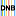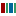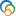The Library of Congress > Linked Data Service > LC Subject Headings (LCSH)

# Multilevel models (Statistics)

• ### Variants

• Hierarchical linear models (Statistics)
• Mixed effects models (Statistics)
• Random coefficient models (Statistics)
• Variance component models (Statistics)

• ### Closely Matching Concepts from Other Schemes

•Gemischtes Modell•Hierarchische Matrix•multilevel modelLabel from public data source Wikidata
•Multilevel models (Statistics)• ### Sources

• found: Work cat.: 00435833: Specification methods for the multilevel model, 2000:p. 1 (a regression model for data with a hierarchical structure; synonyms: random coefficient model, hierarchical linear model, mixed effects model, variance component model)
• found: CompuMath cit. index(multilevel model[s])
• found: LC database, July 18, 2001(in titles: multilevel models)
• notfound: CRC concise encyc. math.;Eisenreich. Mathematik;Encyc. dict. math.;Encyc. math.;Math. subj. classif.

• ### Change Notes

• 2001-07-18: new
• 2001-08-30: revised